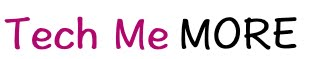To quench our thirst of sharing knowledge about our day to day experience & solution to techincal problems we face in our projects.

Send us an email at diehardtechy@gmail.com

## Monday, December 22, 2014

Problem : Given an unsorted array of size n. Array elements are in range from 1 to n.One number from set {1, 2, …n} is missing and one number occurs twice in array. Find these two numbers.

Approach to solve (Algorithm)

1. Sort the input unsorted Array in ascending order.
2. Run a loop from index 0 to length-1;
2. Find the difference between element at i & i+1 index.
if difference==-1 continue the loop;
else
if(difference==0) //So the Number at i index is repeated
else

if(difference==-2)//So the Number at index[i]+1 is missing.

Solution:

```/**
* Created by DieHardTechy on 22-12-2014.
*/

import java.util.Arrays;

public class ArrayMissingDuplicate {

public static void main(String[] arg) {
//Unsorted Array which contains a duplicate number and a missing number
int unsortedArr[] = {9, 8, 6, 6, 7, 4, 3, 1, 2};
findMissingDuplicate(unsortedArr);
}

public static void findMissingDuplicate(int[] inputArr) {
Arrays.sort(inputArr); //Sorts the input array in ascending order

for (int i = 0; i < inputArr.length - 1; i++) {
if (inputArr[i] - inputArr[i + 1] == -1) {
continue;
} else if (inputArr[i] - inputArr[i + 1] == 0) {
System.out.println("Duplicate number is " + inputArr[i]);
} else if (inputArr[i] - inputArr[i + 1] == -2) {
System.out.println("Missing number is " + (inputArr[i] + 1));
}
}
}

}
```